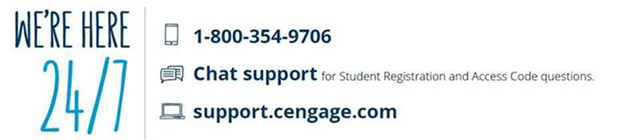Search

AMS 261, Applied Calculus III

Catalog Description: Vector algebra and analytic geometry in 2- and 3-dimensions: multivariable differential calculus and tangent planes; multivariable integral calculus; optimization and Lagrange multipliers; vector calculus including Green's and Stoke's theorems. May not be taken for credit in addition to MAT 203 or 205.

PrerequisitesAMS 161 or MAT 127 or 132 or 142.

4 credits: 3 hours of lecture and 1 hour of recitation

SPRING 2019:

The AMS Department recommends the Cengage Unlimited option ( cengage.com/unlimited ) for students who may enroll in future courses where departments use Cengage Publishing textbooks/eBooks

1.Purchase options

Students may purchase direct from the University bookstore or directly from the publisher, Cengage, via WebAssign
Note:  Students should purchase materials using your Stony Brook email to avoid access issues

Choose one of the following items:
a. Cengage Unlimited \$119.99 (a digital subscription service (think Netflix or Apple Music)
b. WebAssign multi-term code \$125 (full sequence WebAssign calculus, with e-book)
c. WebAssign single term \$100 (one semester WebAssign calculus, with e-book))

Cengage Unlimited Subscription includes:

• WebAssign and e-book access for calculus sequence with a free print rental. PLUS ANY Cengage materials you are using across ALL of your courses AND a library of 20,000 e-books.
• Help yourself get a better grade in other courses with our study guides even if you are not using Cengage.
• Access to Quizlet, a hugely popular mobile and web-based study application that helps students make simple learning tools like flashcards and games.
• Access to Career Center tools, students can build employability skills through career readiness tutorials and create a résumé / portfolio with Pathbrite.

2. How to access your course materials after purchase

3. Technical support************************************************************

AMS 261 IS ALSO OFFERED DURING SUMMER SCHOOL. CHECK THE SUMMER SCHOOL BULLETIN FOR TIMES.

Topics
1. Vector algebra and analytic geometry in two and three dimensions  - 6 hours
2. Multivariate Differential Calculus- partial derivatives and gradients, tangent planes - 6 hours
3. Multivariate Integral Calculus:  double and triple integrals, change of variables and Jacobians, polar coordinates, applications to probability - 10 hours
4. Optimization: maxima and minima, Lagrange multipliers - 6 hours
5 . Vector Calculus: vector-valued functions, curves in space, linear integrals, surface integrals, Green's Theorem, Stoke's Theorem - 10 hours
6. Review and Tests - 4 hours

Learning Outcomes for AMS  261, Applied Calculus III

1.) Demonstrate a firm understanding of the vector algebra and the geometry of two-and three-dimensional space. Specifically students should be able to:
* explain and apply both the geometric and algebraic properties of vectors in two and three dimensions.
* compute dot and cross products, and explain their geometric meaning.
* sketch and interpret vector-valued functions in two and three dimensions.
* differentiate and integrate vector-valued functions.
* explain and apply polar, cylindrical and spherical coordinate systems.

2.) Demonstrate an understanding of scalar functions in several dimensions, and the application of differential and integral calculus to multi-variable functions. Specifically students should be able to:
* describe and sketch curves and surfaces in three-dimensional space.
* compute the partial derivatives of multi-variable functions.
* compute and explain directional derivatives and gradients.
* determine the extreme values of multiple variable functions.
* use Lagrange multipliers to solve constrained optimizations problems.
* solve double- and triple-integrals using iterated integration.
* set up double- and triple-integrations problems in both Cartesian and curvilinear coordinate systems.
* explain and apply the use of Jacobians in solving double- and triple-integrals by coordinate substitution.

3.) Demonstrate a understanding of the fundamental concepts of vector algebra and vector calculus; specifically students should be able to:
* describe and sketch vector fields in two and three dimensions.
* compute and interpret line and surface integrals through scalar or vector fields.
* explain and apply Green’s Theorem.
* explain and apply the Divergence Theorem.
* explain and apply Stokes’ Theorem.

4.) Strengthen ability in communicating and translating of mathematical concepts, models to real world settings:
* present solutions to problems in a clear, well-laid out fashion;
* explain key concepts from the class in written English;
*convert problems described in written English into an appropriate mathematical form;
* convert the mathematical solutions into a written answer.
* use the maple computer program as an aid in solving and visualizing mathematical problems.